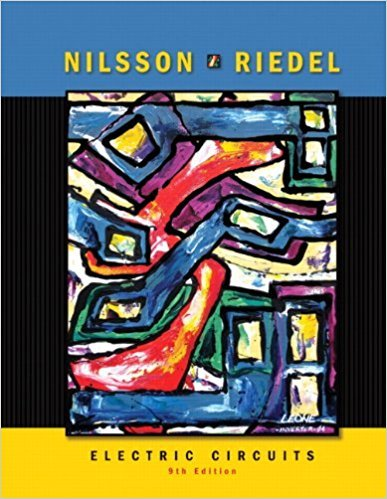×
×

# Assume the input resistance of the op amp in PSPKE Fig. P5.44 is infinite and its outputISBN: 9780136114994 391

## Solution for problem 5.44 Chapter 5

Electric Circuits | 9th Edition

• Textbook Solutions
• 2901 Step-by-step solutions solved by professors and subject experts
• Get 24/7 help from StudySoup virtual teaching assistantsElectric Circuits | 9th Edition

4 5 1 245 Reviews
11
1
Problem 5.44

Assume the input resistance of the op amp in PSPKE Fig. P5.44 is infinite and its output resistance is zero. MULTISIM a) Find v0 as a function of vg and the open-loop gain A. b) What is the value of v0 if vg - 1 V and A = 150? c) What is the value of v0 if vg = 1 V and A - oo? d) How large does A have to be so that v{) is 99% of its value in (c)?

Step-by-Step Solution:
Step 1 of 3

MODULE 4 ARTICLE I. INFERENCE FOR POPULATION MEANS 1) Confidence Intervals a. We can find confidence intervals of two differencesample means 2) Known Population Variances a. Let X and Y be to independent binomial random variables. b. A 100(1-α)% CI for the difference of the two meansfrom each random...

Step 2 of 3

Step 3 of 3

##### ISBN: 9780136114994

Unlock Textbook Solution